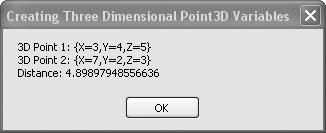# Recipe 6.14. Creating Three-Dimensional Variables

#### Problem

You want to work with three-dimensional coordinates as single entities.

#### Solution

Sample code folder: Chapter 06\ThreePoint

Create a Point3D class that works like the PointF class except that it contains a Z property in addition to X and Y.

#### Discussion

The following class definition is similar to the Point2D class presented in Recipe 6.12:

` Public Class Point3D    Public X As Double    Public Y As Double    Public Z As Double    Public Sub   New(ByVal xPoint As Double, _          ByVal yPoint As Double, ByVal zPoint As Double)       Me.X = xPoint       Me.Y = yPoint       Me.Z = zPoint    End Sub    Public Overrides Function Tostring() As String       Return "{X=" & X & ",Y=" & Y & ",Z=" & Z & "}"    End Function End Class `

The most important modification is the addition of a public Z value for the third dimension. As presented here, the X, Y, and Z properties are all Double precision, but you can easily redefine these to Single if that provides sufficient precision for your calculations, and if you want to save memory when you create large arrays of this data type.

The following code demonstrates the use of some Point3D objects. Notice how the New() function lets you create a Point3D variable with nonzero X, Y, and Z values:

` Dim result As New System.Text.StringBuilder Dim distance As Double Dim point1 As Point3D Dim point2 As Point3D Dim deltaX As Double Dim deltaY As Double Dim deltaZ As Double point1 = New Point3D(3, 4, 5) point2 = New Point3D(7, 2, 3) deltaX = point1.X - point2.X deltaY = point1.Y - point2.Y deltaZ = point1.Z - point2.Z distance = Math.Sqrt(deltaX ^ 2 + deltaY ^ 2 + deltaZ ^ 2) result.AppendLine("3D Point 1: " & point1.ToString()) result.AppendLine("3D Point 2: " & point2.ToString()) result.AppendLine("Distance: " & distance.ToString()) MsgBox(result.ToString()) `

Figure 6-14 shows the results of calculating the distance in space between these two coordinates.

##### Figure 6-14. Manipulating three-dimensional coordinates with a Point3D classSearch for "basic 3D math" on the Web for a variety of explanations and further information about this subject.Visual Basic 2005 Cookbook: Solutions for VB 2005 Programmers (Cookbooks (OReilly))
ISBN: 0596101775
EAN: 2147483647
Year: 2006
Pages: 400

Similar book on Amazon